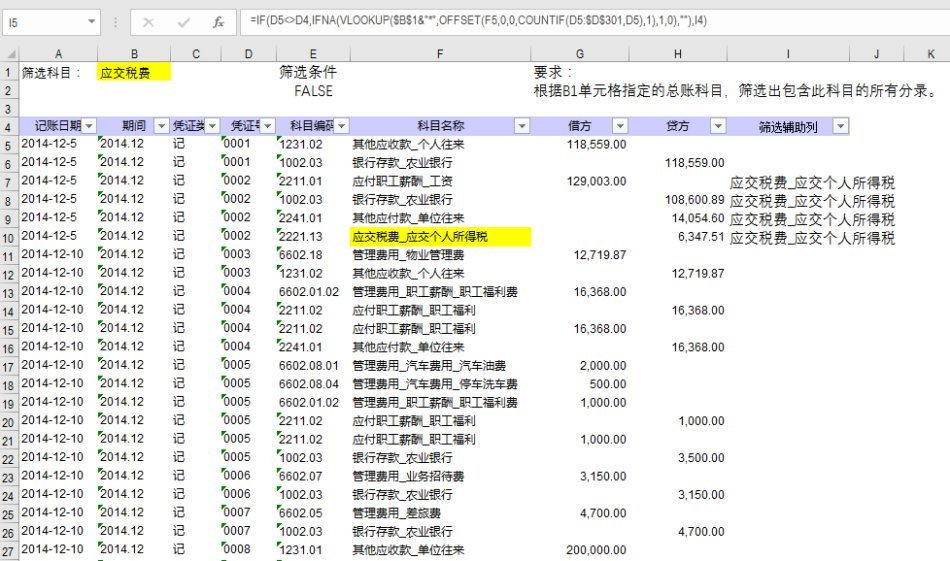# 财务工作常用Excel公式集锦及解析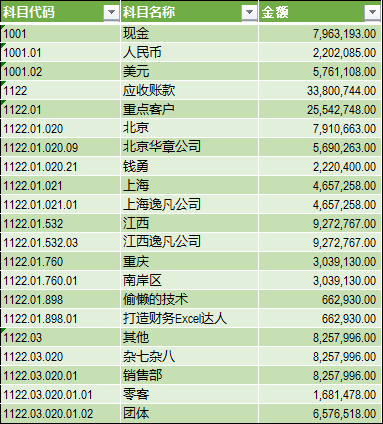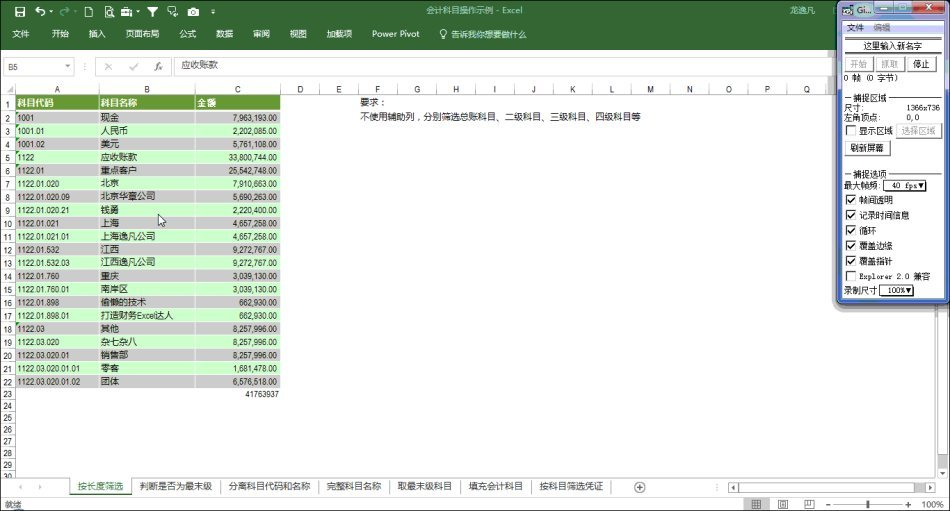“?”是通配符，代表单个字符。所以在筛选搜索框里输入几个问号就代表筛选几个字符的记录，对字母、汉字、数字、字符均有效。我们将此知识点进一步拓展，可以按字符个数求和，比如对所有总账科目（字符长度为四个）进行求和的公式：

=SUMIF(A2:A22,”????”,C2:C22)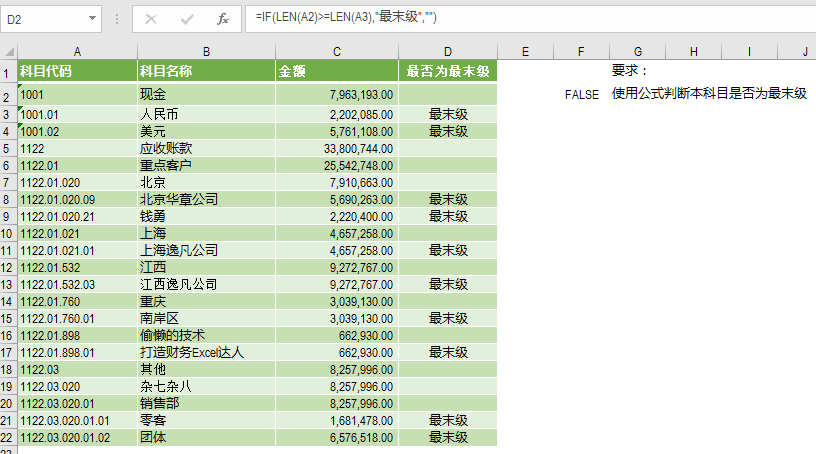=IF(LEN(A2)>=LEN(A3),”最末级”,“”)

=LEN(A2)>=LEN(A3)，再以其为条件筛选，可筛选出大部分符合条件的记录。由于条件公式中的A3按要求应该为\$A\$3，但是如果写成这样，就与需求不符了，故写成A3，这样一来就不符合高级筛选“条件公式中除记录的第一行外的所有其他引用要求是绝对引用”这一条件，因而最后一行未筛选出，存在小小的瑕疵，因而不适合使用高级筛选。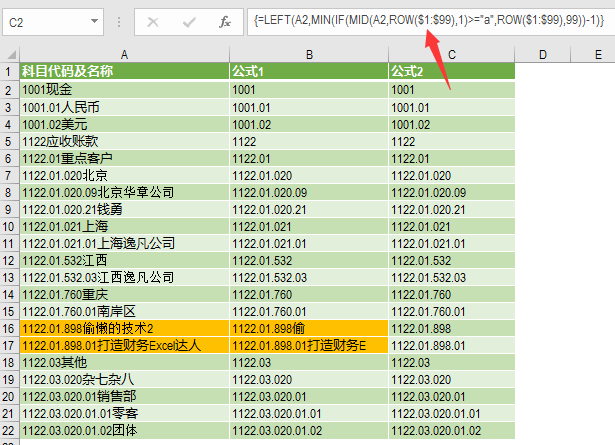=LEFT(A2,MIN(IF(MID(A2,ROW(\$1: \$99),1)>=”a”,ROW(\$1: \$99),99))-1)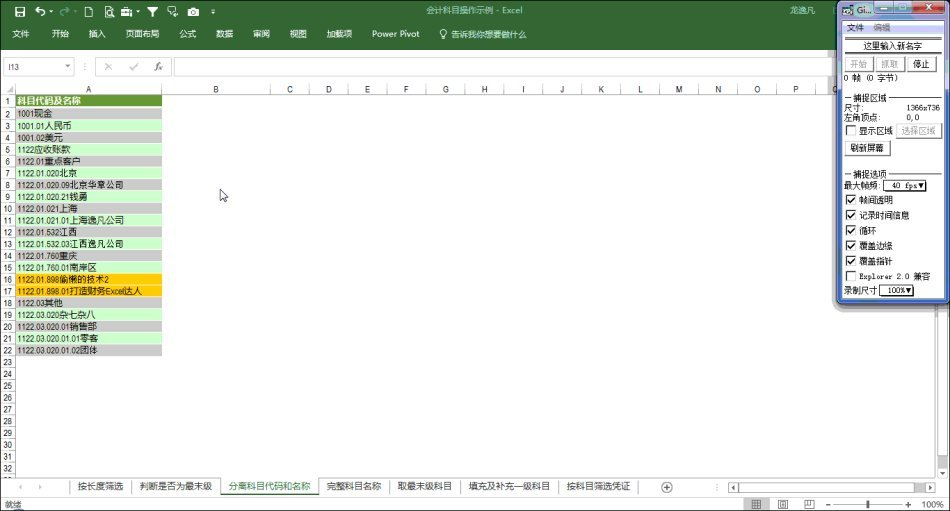MID(A2,ROW(\$1: \$99),1)是依次截止A2单元格的第1个，第2个。。。第99个。截取出的字符如果是字母或汉字，则给其字符所在位置的顺序号，否则，给它的顺序号是99。再用MIN函数来提取第一个字母、汉字的位置。最后用LEFT来截取左边的数字和字符组成的代码。

=RIGHT(A2,LEN(A2)-MIN(IF(MID(A2,ROW(\$1: \$99),1)>=”a”,ROW(\$1: \$99),99))+1)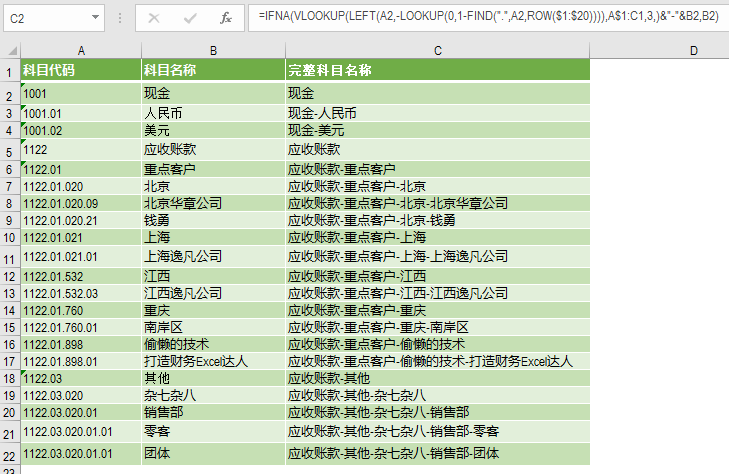=IFNA(VLOOKUP(LEFT(A2,-LOOKUP(0,1-FIND(“.”,A2,ROW(\$1: \$20)))),A\$1:C1,3,)&”-“&B2,B2)

ROW(\$1: \$20)生成1到20的常量数组（假设代码长度都在20以内）。

FIND(“.”,A2,ROW(\$1: \$20))依次从第1位、第2位…第20位开始查找”.”符号。如果没有查找到则出错。此公式将生成一个由错误值及”.”所在位置组成的序列。

1-FIND(“.”,A2,ROW(\$1: \$20))将生成错误值与0,-1,-2….组成的序列。

-LOOKUP(0,1-FIND(“.”,A2,ROW(\$1: \$20)))此公式在序列中查找0，根据其查找原理将返回最后一个”.”前面那个字符的位置。如果对这点还是不太理解，请阅读《深入理解LOOKUP：LOOKUP函数的查找原理》、《公式-LOOKUP(1,-LEFT(A1,ROW(\$1: \$10)))详解》。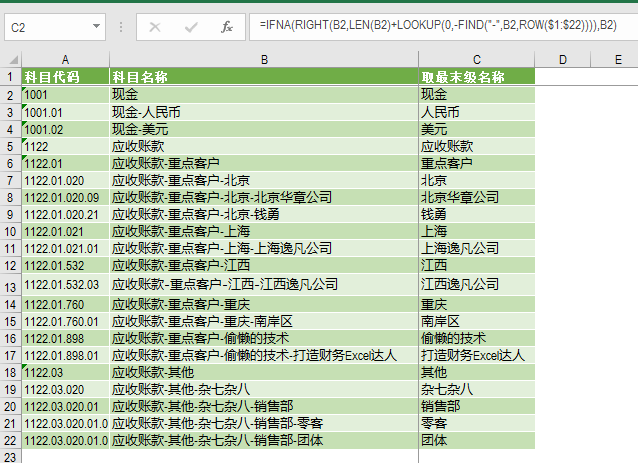=IFNA(RIGHT(B2,LEN(B2)+LOOKUP(0,-FIND(“-“,B2,ROW(\$1: \$22)))),B2)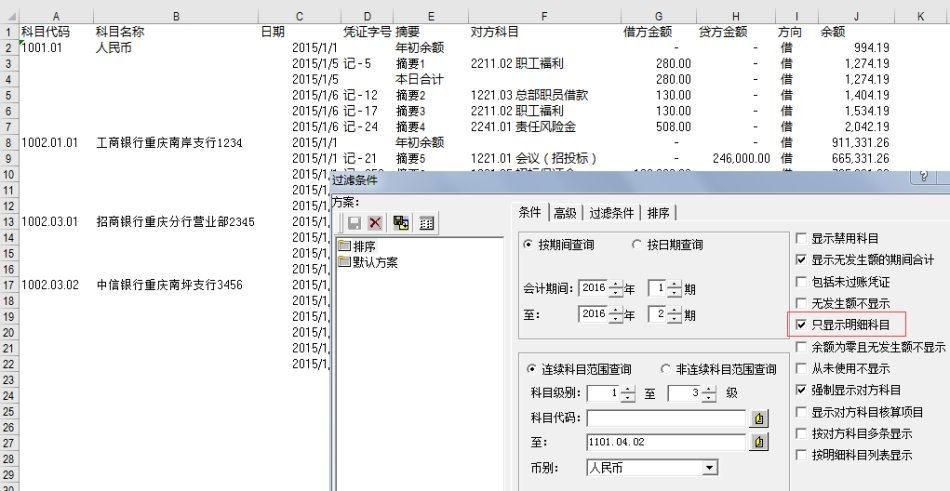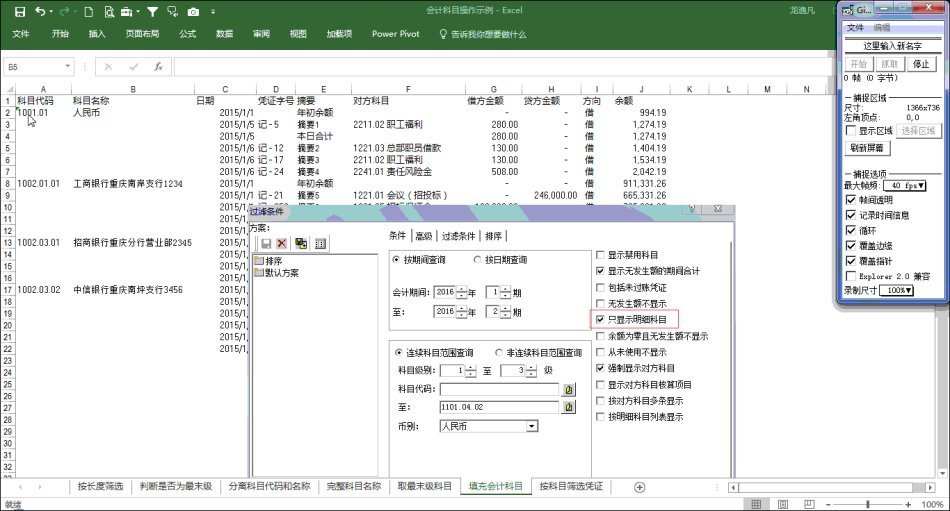F5功能键在Excel中是定位功能的快捷键，定位功能是精确制导的武器，它可根据单元格的属性来选择单元格。常用于选择数字单元格、公式单元格、空白单元格、没有隐藏的单元格。

=IF(D7<>D6,VLOOKUP(\$B\$1&”*”,OFFSET(F7,0,0,COUNTIF(D7: \$D\$301,D7),1),1,0),I6)

=IF(D5<>D4,IFNA(VLOOKUP(\$B\$1&”*”,OFFSET(F5,0,0,COUNTIF(D5: \$D\$301,D5),1),1,0),””),I4)